# Triangle Inequality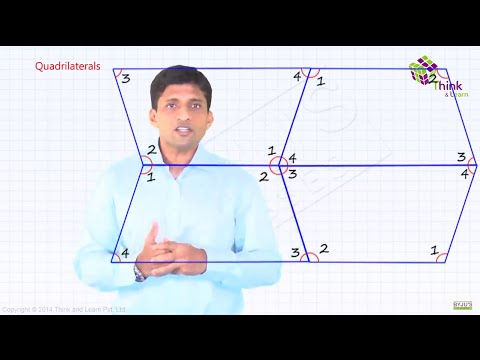Triangle is a 3 sided polygon.  A triangle has three sides and three angles. The three sides and three angles share an important relationship. Let us discuss about the relationship and equality and inequality of triangle, through an activity.

Activity: On a paper mark two points Y and Z and join them to form a straight line. Mark another point X outside the line lying on the same plane of the paper. Join XY as shown.

Now mark another point X’ on the line segment XY, join X’Z. Similarly mark X’’ and join X’’Z with dotted lines as shown.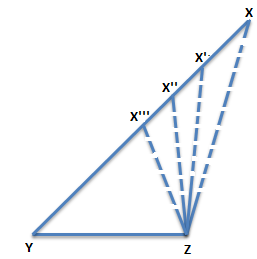From the above figure we can easily deduce that if we keep on decreasing the length of side XY such that XY> X’Y> X’’Y> X’’’Y the angle opposite to side XY also decreases i.e. ∠XZY >∠X’ZY >∠X’’ZY >∠X’’’ZY. Thus from the above activity we can infer that if we keep on increasing one side of a triangle then the angle opposite to it increases .

Now let us try out another activity.

Activity: Draw 3 scalene triangles on a sheet of paper as shown.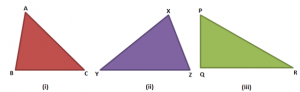Let us consider fig. (i). In ∆ABC, AC is the longest side and AB is the shortest. We observe that ∠B is the largest in measure and ∠C is the smallest. Similarly in ∆XYZ, XY is the largest side and XZ is the smallest and ∠Z is the largest in measure and ∠Y is the smallest. In the last figure also same kind of pattern is followed i.e. side PR is largest and so is the ∠Q opposite to it. This can be stated as a triangles theorems:

### Triangle Inequality Theorem

Theorem 1: In a triangle, the side opposite to the largest side is greatest in measure.

The converse of the above theorem is also true according to which in a triangle the side opposite to greater angle is the longest side of the triangle.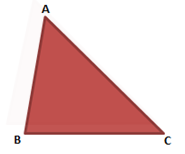In above fig., since AC is the longest side, largest angle in the triangle is ∠B.

Another theorem which follows can be stated as:

Theorem 2: The sum of lengths of any two sides of a triangle is greater than the length of its third side.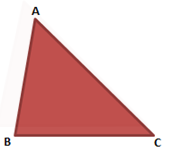According to triangle inequality, AB + BC > AC